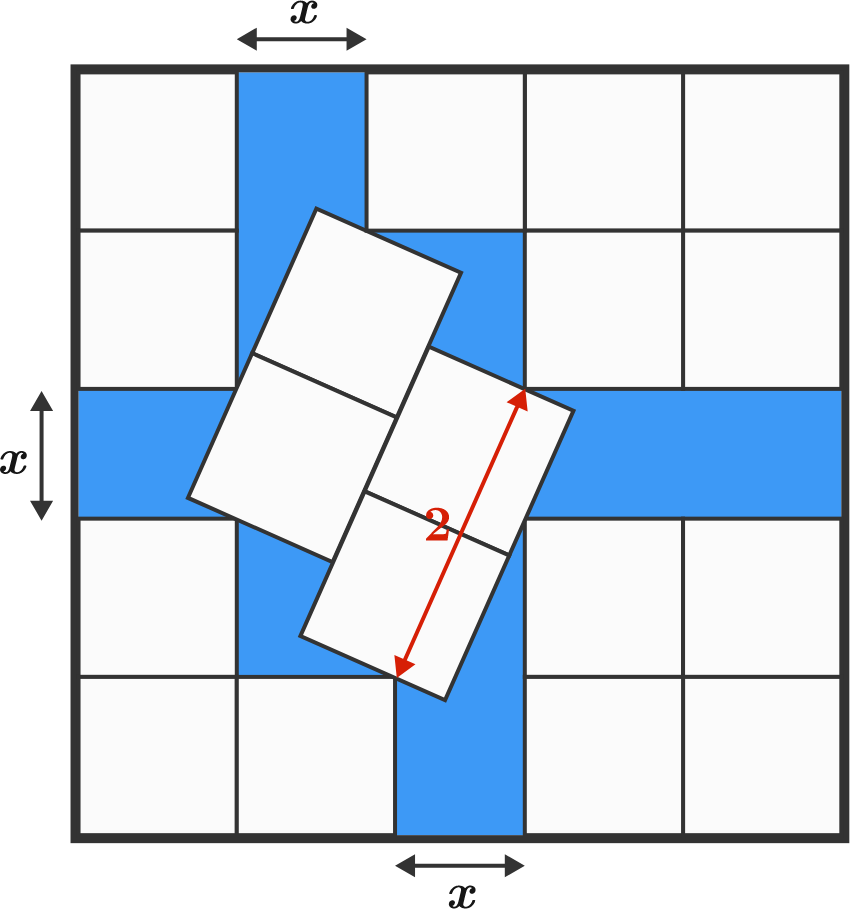# Boxed in a Box

Geometry Level 3

There are 18 white squares of side length 1 packed inside a larger square.The shaded area is equal to $\dfrac{A\sqrt{A}}{2}-B$, where $A$ is a prime number.

Find $A+B$.

×